# Equation

Eequation f(x) = 0 has roots x1 = 64, x2 = 100, x3 = 25, x4 = 49. How many roots have equation f(x2) = 0 ?

Correct result:

n =  8

#### Solution: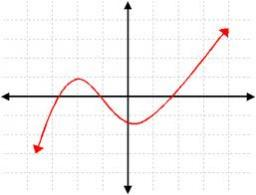We would be pleased if you find an error in the word problem, spelling mistakes, or inaccuracies and send it to us. Thank you!Tips to related online calculators
Looking for help with calculating roots of a quadratic equation?
Do you have a linear equation or system of equations and looking for its solution? Or do you have quadratic equation?

## Next similar math problems:

• RootsDetermine the quadratic equation absolute coefficient q, that the equation has a real double root and the root x calculate: ?Which of the points belong function f:y= 2x2- 3x + 1 : A(-2, 15) B (3,10) C (1,4)Find the roots of the quadratic equation: 3x2-4x + (-4) = 0.
• Function 3Function f(x)=a(x-r)(x-s) the graph of the function has x- intercept at (-4, 0) and (2, 0) and passes through the point (-2,-8). Find constant a, r, s.
• Logarithmic equation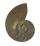Solve equation: log33(3x + 21) = 0
• Roots and coefficient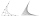In the equation 2x ^ 2 + bx-9 = 0 is one root x1 = -3/2. Determine the second root and the coefficient b.
• Equation with abs value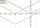How many solutions has the equation ? in the real numbers?
• VariableFind variable P: PP plus P x P plus P = 160
• Reciprocal equation 2Solve this equation: x + 5/x - 6 = 4/11
• EquationEquation ? has one root x1 = 8. Determine the coefficient b and the second root x2.
• Solve 3Solve quadratic equation: (6n+1) (4n-1) = 3n2
• Reciprocal value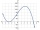How do I calculate a number x that is 9 greater than its reciprocal (1/x)?
• AsymptoteWhat is the vertical asymptote of ?
• DiscriminantDetermine the discriminant of the equation: ?
• Square root 2If the square root of 3m2 +22 and -x = 0, and x=7, what is m?
• Equation 23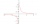Find value of unknown x in equation: x+3/x+1=5 (problem finding x)
• Evaluation of expressionsIf a2-3a+1=0, find (i)a2+1/a2 (ii) a3+1/a3